# RD Sharma Solutions For Class 7 Maths Chapter 17 Constructions Exercise 17.5

In this exercise, we shall discuss about the RHS triangle construction and steps involved in them. The primary objective is to help students improve their problem-solving abilities, which in turn, helps in boosting their confidence level to achieve high marks in their exams. Students can refer and download RD Sharma Solutions for Class 7 Maths Exercise 17.5 Chapter 17 Constructions. These solutions are formulated by BYJU’S expert team in Maths.

## Download the PDF of RD Sharma Solutions For Class 7 Maths Chapter 17 – Constructions Exercise 17.5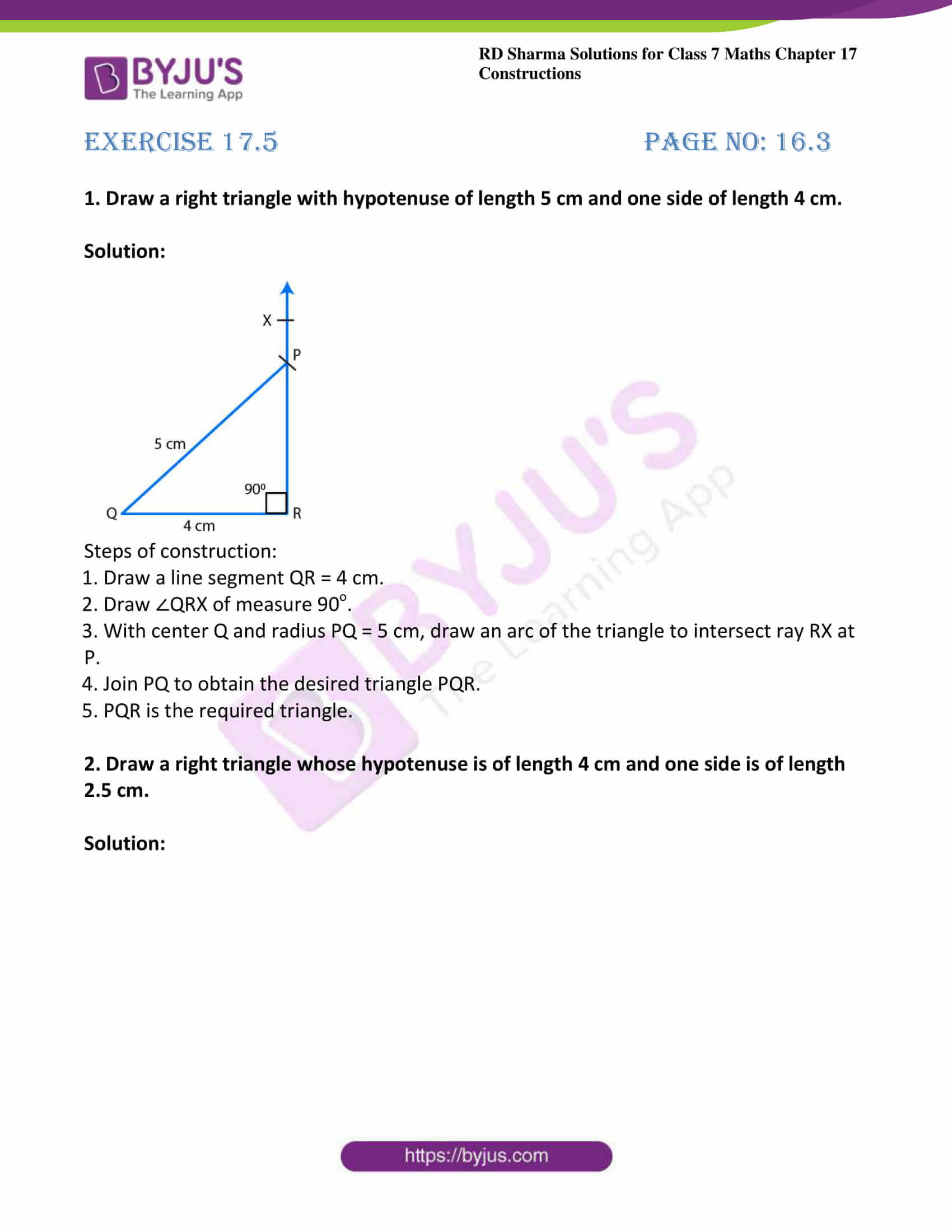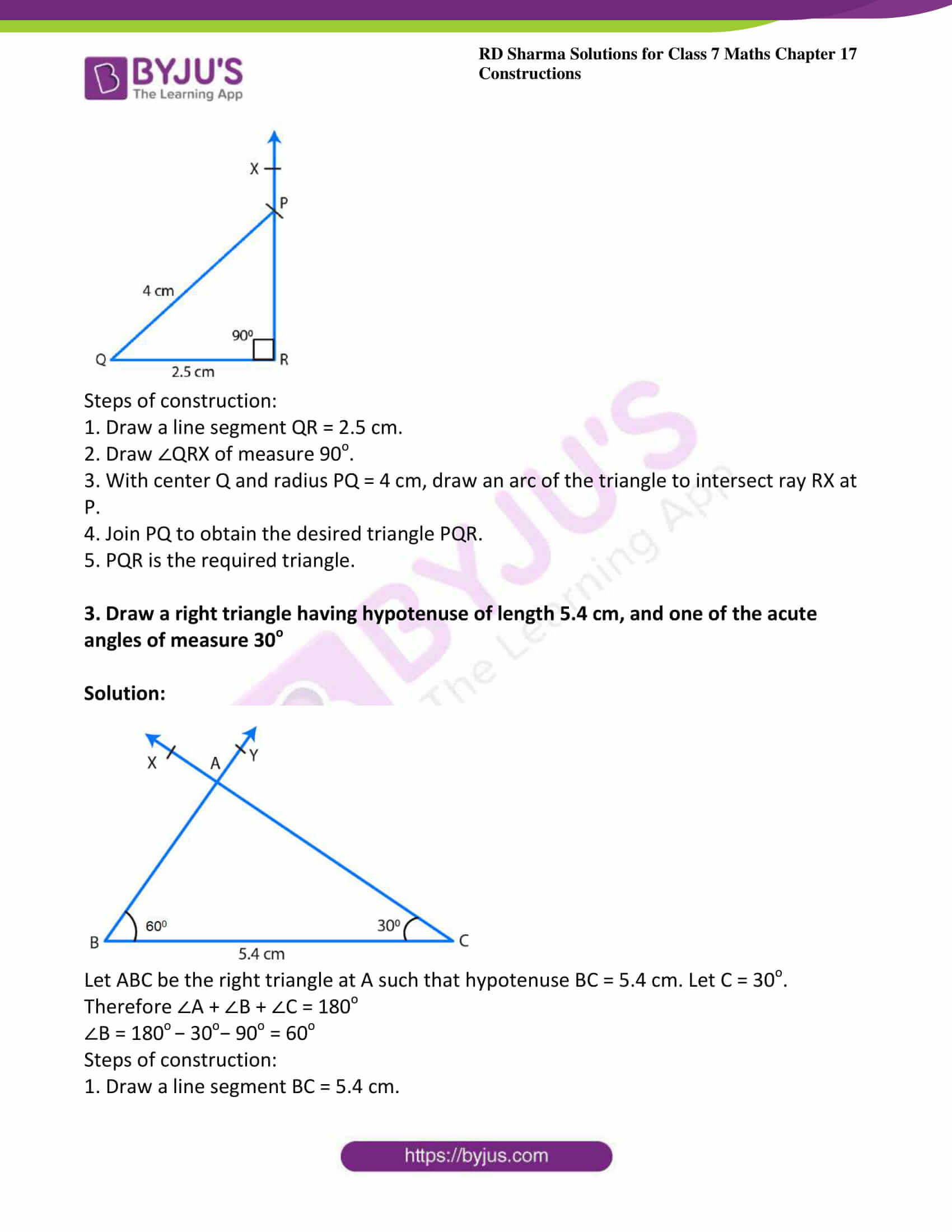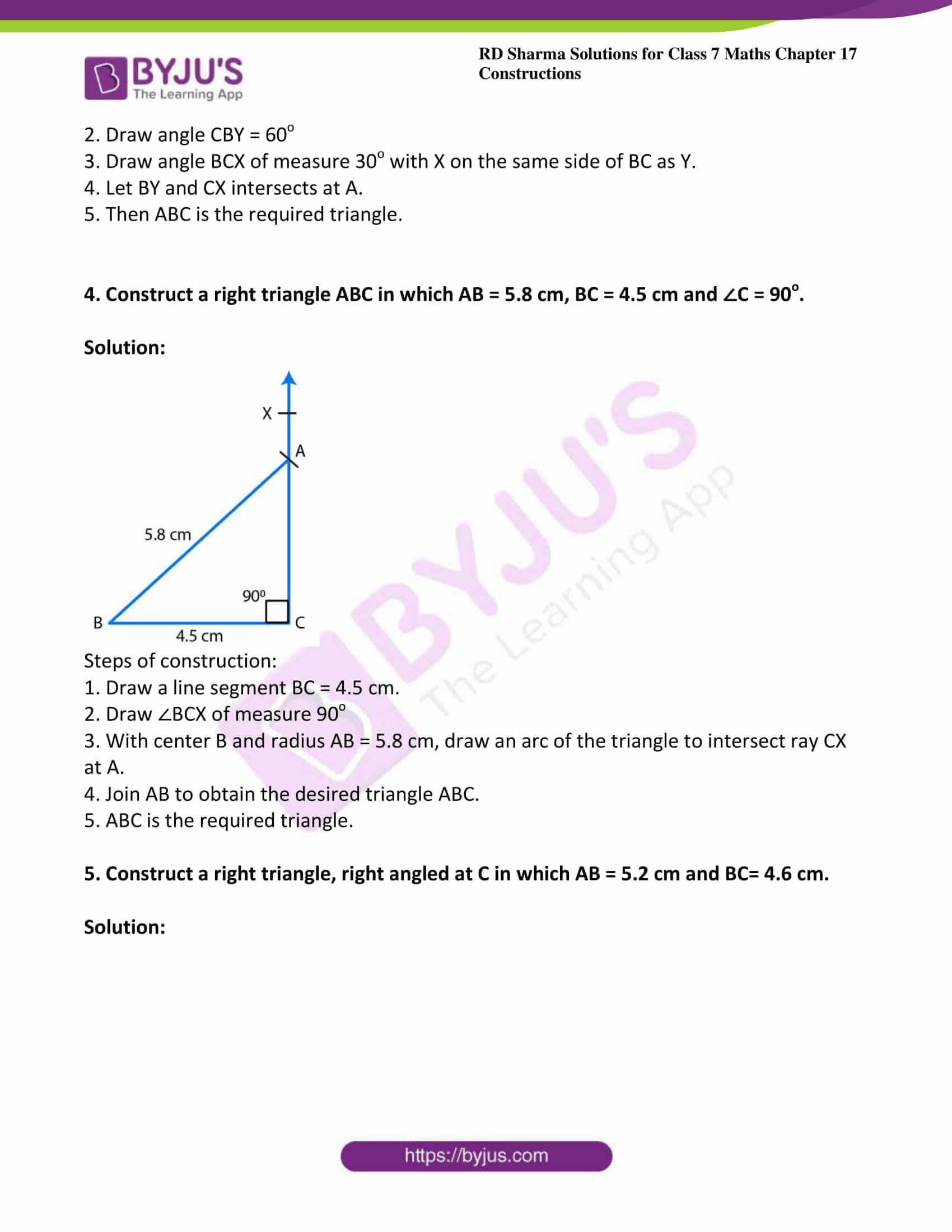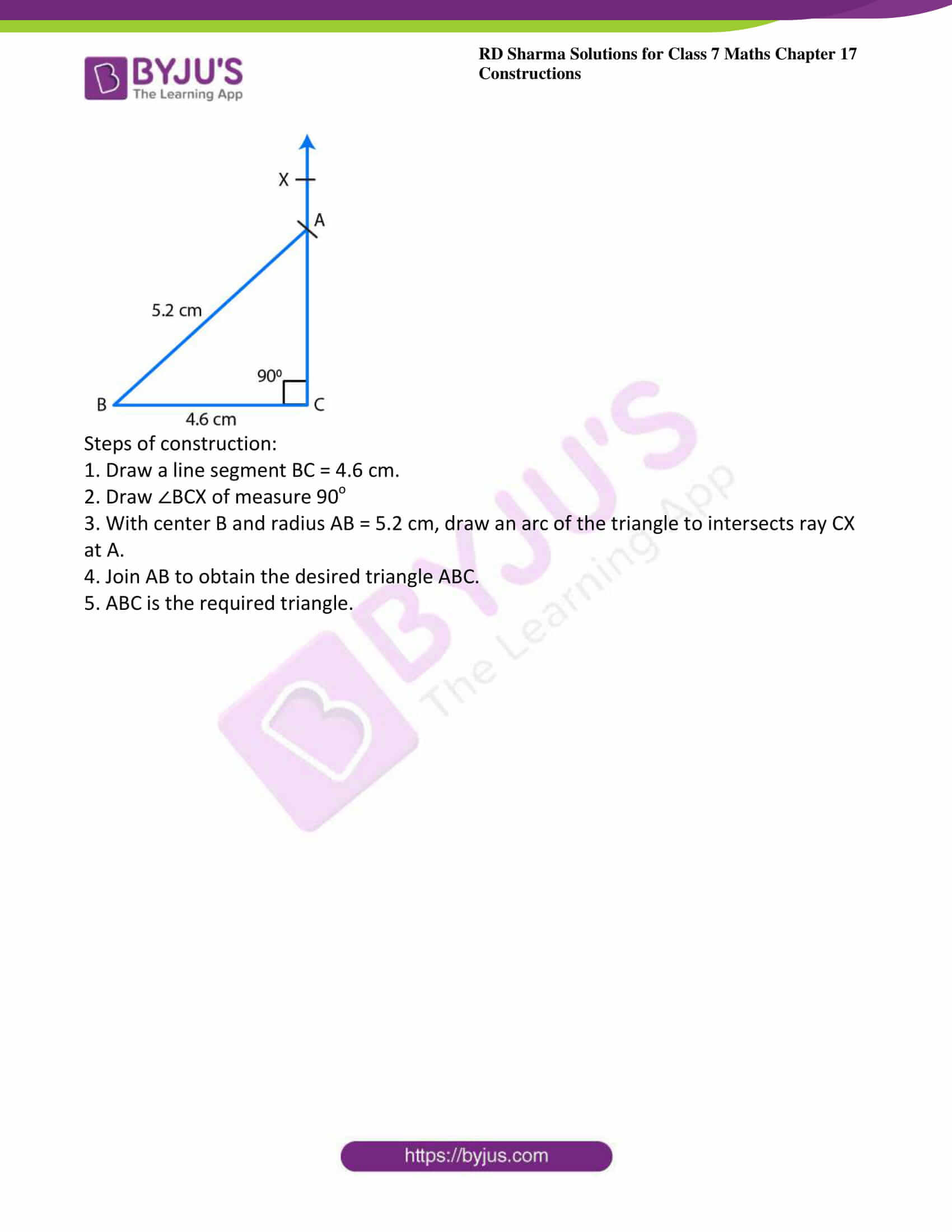### Access answers to Maths RD Sharma Solutions For Class 7 Chapter 17 – Constructions Exercise 17.5

Exercise 17.5 Page No: 16.3

1. Draw a right triangle with hypotenuse of length 5 cm and one side of length 4 cm.

Solution: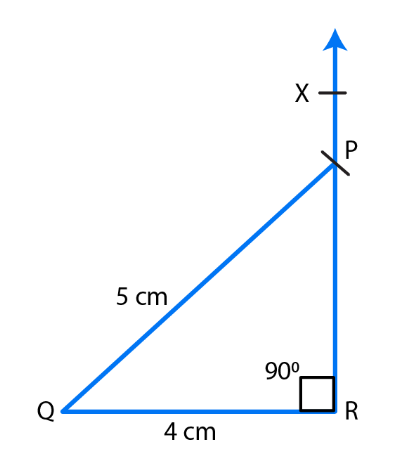Steps of construction:

1. Draw a line segment QR = 4 cm.

2. Draw ∠QRX of measure 90o.

3. With center Q and radius PQ = 5 cm, draw an arc of the triangle to intersect ray RX at P.

4. Join PQ to obtain the desired triangle PQR.

5. PQR is the required triangle.

2. Draw a right triangle whose hypotenuse is of length 4 cm and one side is of length 2.5 cm.

Solution: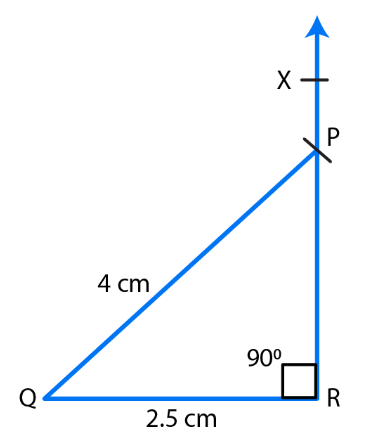Steps of construction:

1. Draw a line segment QR = 2.5 cm.

2. Draw ∠QRX of measure 90o.

3. With center Q and radius PQ = 4 cm, draw an arc of the triangle to intersect ray RX at P.

4. Join PQ to obtain the desired triangle PQR.

5. PQR is the required triangle.

3. Draw a right triangle having hypotenuse of length 5.4 cm, and one of the acute angles of measure 30o

Solution: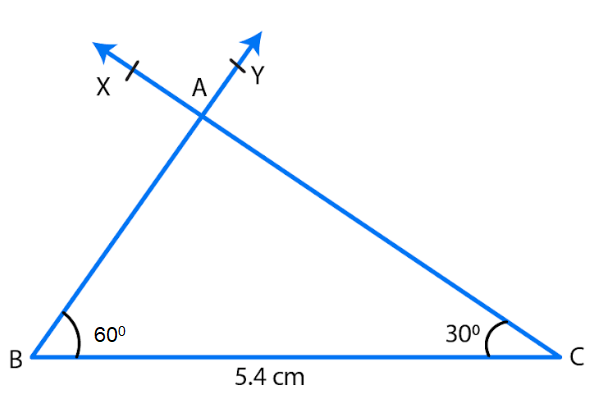Let ABC be the right triangle at A such that hypotenuse BC = 5.4 cm. Let C = 30o.

Therefore ∠A + ∠B + ∠C = 180o

∠B = 180o − 30o− 90o = 60o

Steps of construction:

1. Draw a line segment BC = 5.4 cm.

2. Draw angle CBY = 60o

3. Draw angle BCX of measure 30o with X on the same side of BC as Y.

4. Let BY and CX intersects at A.

5. Then ABC is the required triangle.

4. Construct a right triangle ABC in which AB = 5.8 cm, BC = 4.5 cm and ∠C = 90o.

Solution: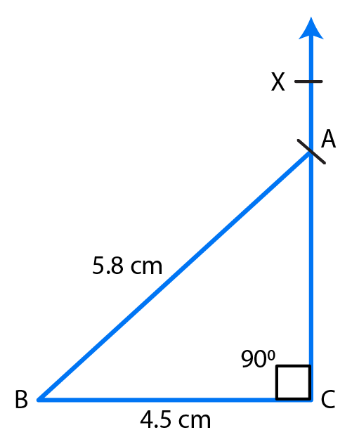Steps of construction:

1. Draw a line segment BC = 4.5 cm.

2. Draw ∠BCX of measure 90o

3. With center B and radius AB = 5.8 cm, draw an arc of the triangle to intersect ray CX at A.

4. Join AB to obtain the desired triangle ABC.

5. ABC is the required triangle.

5. Construct a right triangle, right angled at C in which AB = 5.2 cm and BC= 4.6 cm.

Solution: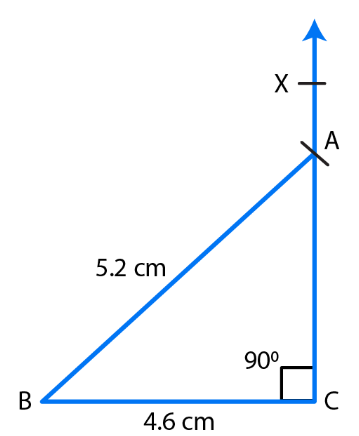Steps of construction:

1. Draw a line segment BC = 4.6 cm.

2. Draw ∠BCX of measure 90o

3. With center B and radius AB = 5.2 cm, draw an arc of the triangle to intersects ray CX at A.

4. Join AB to obtain the desired triangle ABC.

5. ABC is the required triangle.